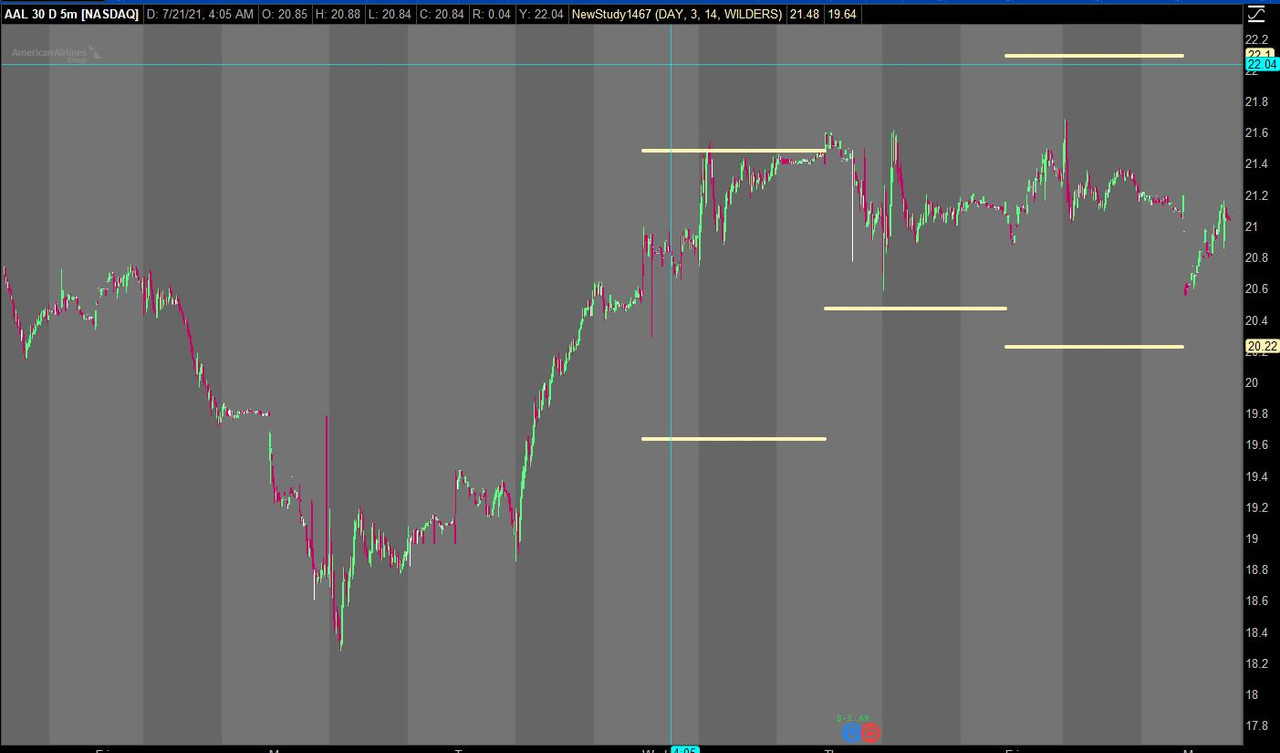# Limiting plot

#### KingsOp

##### New member
In the following code I have 2 plots to indicate the ATR high and low based on the current candle projected into the next candles timeframe. I mostly use this on a day timeframe so I know what a move based on yesterday's close will look like if it is greater than average. The problem is it also shows every day up to that point so there are a LOT of dots (that's what I have them drawn as) on the chart. I'd like to be able to limit the number of dots from the past showing. Hope I'm explaining myself well. So say I only want to see the past three days worth of data, how do I limit the plot to those kind of parameters instead of for the whole history of the chart?

Code:
``````input time = AggregationPeriod.DAY;

#hint time: enter the desired chart timeframe

input length = 14;

#hint length: enter the desired average length

input averageType = AverageType.WILDERS;

#hint averageType: enter the desired average calculation method

def ATR = MovingAverage(averageType, TrueRange(high(period = time), close(period = time), low(period = time)), length);

def ATRUP = close(period = time) + ATR;

def ATRDN = close(period = time) - ATR;

plot UP = ATRUP;

plot DN = ATRDN;

UP.SetDefaultColor(GetColor(8));

DN.SetDefaultColor(GetColor(8));

#hint: This indicator will plot the ATR levels for the given chart timeframe projected from the close of the last bar onto the next bar. Designed intentionally for the DAY timeframe but will work in any timeframe.``````

In the following code I have 2 plots to indicate the ATR high and low based on the current candle projected into the next candles timeframe. I mostly use this on a day timeframe so I know what a move based on yesterday's close will look like if it is greater than average. The problem is it also shows every day up to that point so there are a LOT of dots (that's what I have them drawn as) on the chart. I'd like to be able to limit the number of dots from the past showing. Hope I'm explaining myself well. So say I only want to see the past three days worth of data, how do I limit the plot to those kind of parameters instead of for the whole history of the chart?

Code:
``````input time = AggregationPeriod.DAY;

#hint time: enter the desired chart timeframe

input length = 14;

#hint length: enter the desired average length

input averageType = AverageType.WILDERS;

#hint averageType: enter the desired average calculation method

def ATR = MovingAverage(averageType, TrueRange(high(period = time), close(period = time), low(period = time)), length);

def ATRUP = close(period = time) + ATR;

def ATRDN = close(period = time) - ATR;

plot UP = ATRUP;

plot DN = ATRDN;

UP.SetDefaultColor(GetColor(8));

DN.SetDefaultColor(GetColor(8));

#hint: This indicator will plot the ATR levels for the given chart timeframe projected from the close of the last bar onto the next bar. Designed intentionally for the DAY timeframe but will work in any timeframe.``````

Try thisRuby:
``````input time = AggregationPeriod.DAY;

#hint time: enter the desired chart timeframe

#Plot Limiter
input plotlimiter = 3;
def periods     = close(period = time);
def periodCount = CompoundValue(1, if periods then periodCount + 1 else periodCount, 0);
def thisperiod  = (HighestAll(periodCount) - periodCount + 1) ;
#End Plot Limiter

input length = 14;

#hint length: enter the desired average length

input averageType = AverageType.WILDERS;

#hint averageType: enter the desired average calculation method

def ATR = MovingAverage(averageType, TrueRange(high(period = time), close(period = time), low(period = time)), length);

def ATRUP = close(period = time) + ATR;

def ATRDN = close(period = time) - ATR;

plot UP = if thisperiod > plotlimiter then double.nan else ATRUP;

plot DN = if thisperiod > plotlimiter then double.nan else ATRDN;

UP.SetDefaultColor(GetColor(8));

DN.SetDefaultColor(GetColor(8));

#hint: This indicator will plot the ATR levels for the given chart timeframe projected from the close of the last bar onto the next bar. Designed intentionally for the DAY timeframe but will work in any timeframe.``````

•KingsOp

### Not the exact question you're looking for?

87k+ Posts
282 Online## The Market Trading Game Changer

Join 2,500+ subscribers inside the useThinkScript VIP Membership Club
• Exclusive indicators
• Proven strategies & setups
• Private Discord community
• Exclusive members-only content
• 1 full year of unlimited support

What is useThinkScript?

useThinkScript is the #1 community of stock market investors using indicators and other tools to power their trading strategies. Traders of all skill levels use our forums to learn about scripting and indicators, help each other, and discover new ways to gain an edge in the markets.

How do I get started?

We get it. Our forum can be intimidating, if not overwhelming. With thousands of topics, tens of thousands of posts, our community has created an incredibly deep knowledge base for stock traders. No one can ever exhaust every resource provided on our site.

If you are new, or just looking for guidance, here are some helpful links to get you started.

What are the benefits of VIP Membership?